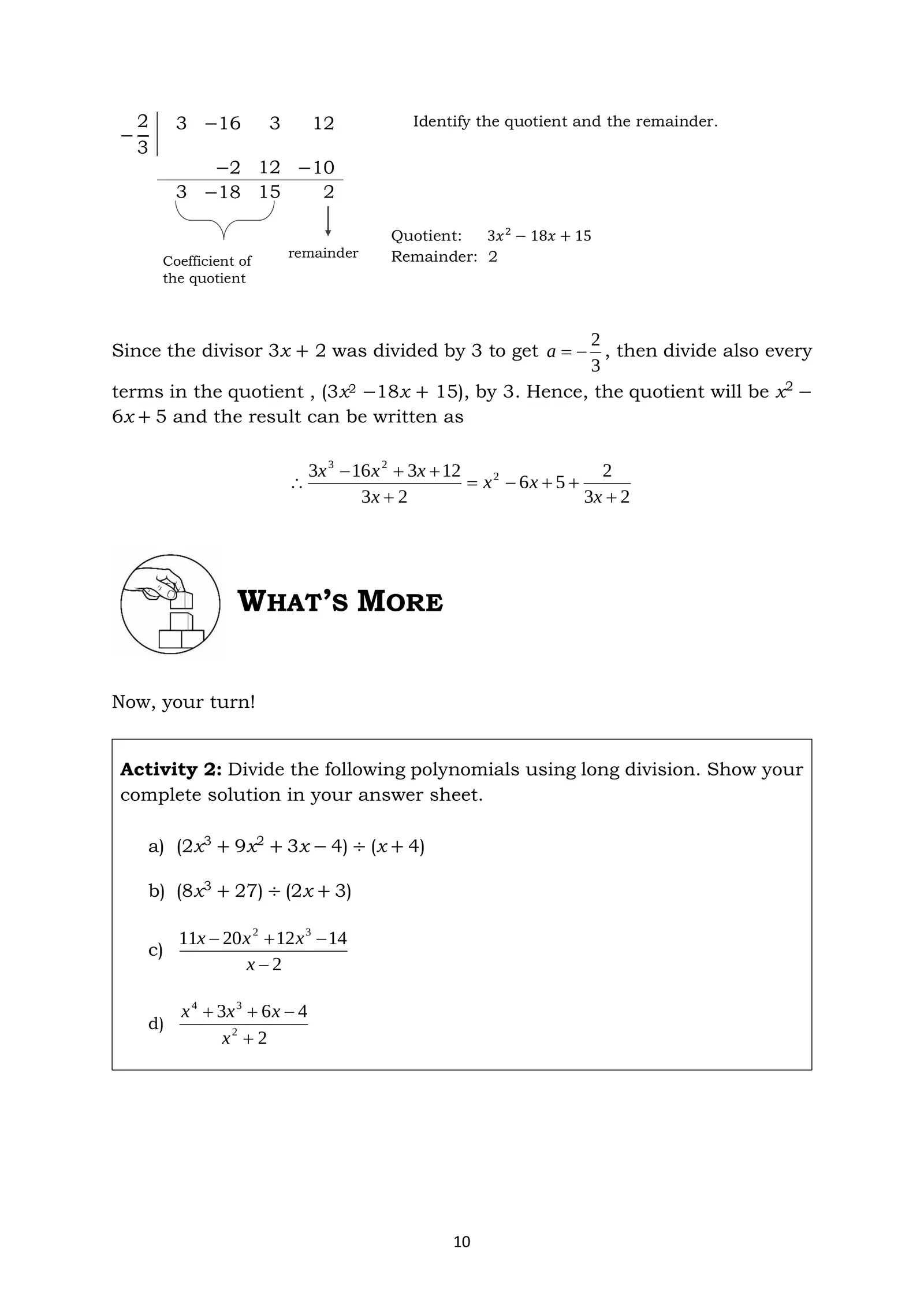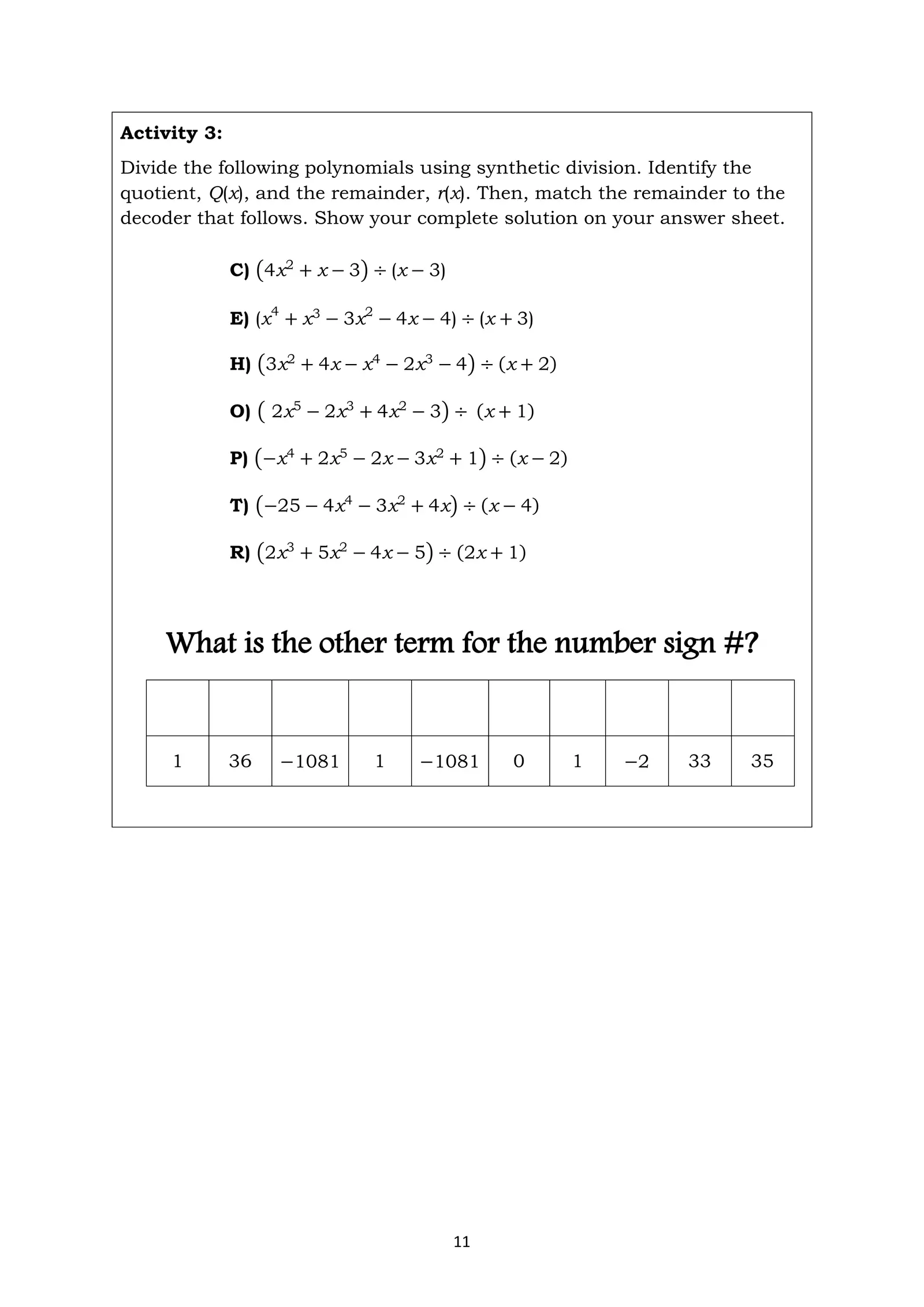# Math10 Q1 Week 8 Activities

Created
Best for live in-class or video conferencing lessonsStart teacher-led lessonActivity 3: Divide the following polynomials using synthetic division. Identify the quotient, Q(x), and the remainder, r(x). Then, match the remainder to the decoder that follows. Show your complete solution on your answer sheet. C) (4x2 + x − 3) ÷ (x − 3) E) (x4 + x3 − 3x2 − 4x − 4) ÷ (x + 3) H) (3x2 + 4x − x4 − 2x3 − 4) ÷ (x + 2ሻ O) ( 2x5 − 2x3 + 4x2 − 3) ÷ (x + 1ሻ P) (−x4 + 2x5 − 2x − 3x2 + 1) ÷ (x − 2ሻ T) (−25 − 4x4 − 3x2 + 4x) ÷ (x − 4ሻ R) (2x3 + 5x2 − 4x − 5) ÷ (2x + 1ሻ What is the other term for the number sign #? 1 36 −1081 1 −1081 0 1 −2 33 35 11

Name:
Date:
Answer only Activity 2 and 3.
Type your solutions in the box.
a)
c)
b)
d)WHAT I HAVE LEARNED Let us sum up what we have learned in this module. Activity 4. Perform what is asked. A) Fill in the blank with the correct term. When dividing polynomials, we express the result in the following form: P(x) r(x) = Q(xሻ + d(x) d(x) Where P(x) is the 1 , d(x) ≠ 0 is the 2 , Q(x) is the 3 , and r(x) is the 4 . B) Use the synthetic division below to find the following: 2 2 0 0 −1 −36 a. Dividend: 4 8 16 30 b. Divisor: 2 4 8 15 −6 c. Remainder: d. Quotient: 12

Direction: Type the letter of inside the box in ALL CAPS.
Direction: Type your solutions in the box.
C
E
H
O
P
T
R